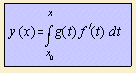# derivative and integral

## main

Given a curve C1, the derivative is the curve C2 defined by the derivation of the y component to the x component. So the y component of C2 gives the slope of C1.
When the curve is not smooth, or not a function, special effects can occur.

Suppose C1 is given by (x, y) = (f(t), g(t)) then C2 is given by y(x) = g '(x) / f '(x).

When the curve is formulated in polar coordinates, a polar pendant of the derivative is: dr/dφ. This is then the slope in r as function of φ.

The reverse of differentiation is the operation of integration:
Given a curve C1, the integral is the curve C2 defined by the integration of the y component to the x component. So the y component of C2 gives the cumulated area between C1 and the x-as.

When C1 is given by (x, y) = (f(t), g(t)), then C2 has the form:In polar coordinates, integration is over a sector between angles φ0 and φ.